SLOS313C December   2000  – March 2016

PRODUCTION DATA.

1. Features
2. Applications
3. Description
4. Revision History
5. Device Comparison Table
6. Pin Configuration and Functions
7. Specifications
8. Parameter Measurement Information
9. Detailed Description
10. 10Application and Implementation
1. 10.1 Application Information
2. 10.2 Typical Application
11. 11Power Supply Recommendations
12. 12Layout
13. 13Device and Documentation Support
14. 14Mechanical, Packaging, and Orderable Information

#### Package Options

Refer to the PDF data sheet for device specific package drawings

• D|8
• DGN|8
• DGN|8

## 7 Specifications

### 7.1 Absolute Maximum Ratings

over operating free-air temperature range (unless otherwise noted) (1)
MIN MAX UNIT
VDD Supply voltage 6 V
VI Input voltage –0.3 VDD + 0.3 V
Continuous total power dissipation Internally Limited
TJ Operating junction temperature –40 150 °C
Lead temperature 1,6 mm (1/16 inch) from case for 10 seconds 260 °C
Tstg Storage temperature –65 150 °C
(1) Stresses beyond those listed under Absolute Maximum Ratings may cause permanent damage to the device. These are stress ratings only, which do not imply functional operation of the device at these or any other conditions beyond those indicated under Recommended Operating Conditions. Exposure to absolute-maximum-rated conditions for extended periods may affect device reliability.

### 7.2 ESD Ratings

VALUE UNIT
V(ESD) Electrostatic discharge Human-body model (HBM), per ANSI/ESDA/JEDEC JS-001(1) ±2000 V
Charged-device model (CDM), per JEDEC specification JESD22-C101(2) ±1500
(1) JEDEC document JEP155 states that 500-V HBM allows safe manufacturing with a standard ESD control process.
(2) JEDEC document JEP157 states that 250-V CDM allows safe manufacturing with a standard ESD control process.

### 7.3 Recommended Operating Conditions

MIN MAX UNIT
VDD Supply voltage 2.5 5.5 V
TA Operating free-air temperature –40 85 °C
VIH High-level input voltage (SHUTDOWN) 60% × VDD V
VIL Low-level input voltage (SHUTDOWN) 25% × VDD V

### 7.4 Thermal Information

THERMAL METRIC(1) TPA6111A2 UNIT
D (SOIC) DGN (MSOP)
8 PINS 8 PINS
RθJA Junction-to-ambient thermal resistance 114.7 55.9 °C/W
RθJC(top) Junction-to-case (top) thermal resistance 59.0 47.3 °C/W
RθJB Junction-to-board thermal resistance 54.9 36.4 °C/W
ψJT Junction-to-top characterization parameter 14.2 2.3 °C/W
ψJB Junction-to-board characterization parameter 54.4 36.2 °C/W
RθJC(bot) Junction-to-case (bottom) thermal resistance 9.2 °C/W

### 7.5 DC Electrical Characteristics, VDD = 3.3 V

at VDD = 3.3 V, TA = 25°C (unless otherwise noted)
PARAMETER TEST CONDITIONS MIN TYP MAX UNIT
VOO Output offset voltage 10 mV
PSRR Power supply rejection ratio VDD = 3.2 V to 3.4 V 70 dB
IDD Supply current SHUTDOWN (pin 5) = 0 V 1.5 3 mA
IDD(SD) Supply current in shutdown mode SHUTDOWN (pin 5) = VDD 1 10 µA
Zi Input impedance > 1

### 7.6 AC Operating Characteristics, VDD = 3.3 V

VDD = 3.3 V, TA = 25°C, RL = 16 Ω
PARAMETER TEST CONDITIONS MIN TYP MAX UNIT
PO Output power (each channel) THD ≤ 0.1%, f = 1 kHz 60 mW
THD+N Total harmonic distortion + noise PO = 40 mW, 20 Hz – 20 kHz 0.4%
BOM Maximum output power BW G = 20 dB, THD < 5% > 20 kHz
Phase margin Open-loop 96°
Supply ripple rejection f = 1 kHz, C(BYP) = 0.47 µF 71 dB
Channel/channel output separation f = 1 kHz, PO = 40 mW 89 dB
SNR Signal-to-noise ratio PO = 50 mW, AV = 1 100 dB
Vn Noise output voltage AV = 1 11 µV(rms)

### 7.7 DC Electrical Characteristics, VDD = 5.5 V

at VDD = 5.5 V, TA = 25°C
PARAMETER TEST CONDITIONS MIN TYP MAX UNIT
VOO Output offset voltage 10 mV
PSRR Power supply rejection ratio VDD = 4.9 V to 5.1 V 70 dB
IDD Supply current SHUTDOWN (pin 5) = 0 V 1.6 3.2 mA
IDD(SD) Supply current in shutdown mode SHUTDOWN (pin 5) = VDD 1 10 µA
|IIH| High-level input current (SHUTDOWN) VDD = 5.5 V, VI = VDD 1 µA
|IIL| Low-level input current (SHUTDOWN) VDD = 5.5 V, VI = 0 V 1 µA
Zi Input impedance > 1

### 7.8 AC Operating Characteristics, VDD = 5.5 V

VDD = 5 V, TA = 25°C, RL = 6 Ω
PARAMETER TEST CONDITIONS MIN TYP MAX UNIT
PO Output power (each channel) THD ≤ 0.1%, f = 1 kHz 150 mW
THD+N Total harmonic distortion + noise PO = 100 mW, 20 Hz – 20 kHz 0.6%
BOM Maximum output power BW G = 20 dB, THD < 5% > 20 kHz
Phase margin Open-loop 96°
Supply ripple rejection ratio f = 1 kHz, C(BYP) = 0.47 µF 61 dB
Channel/channel output separation f = 1 kHz, PO = 100 mW 90 dB
SNR Signal-to-noise ratio PO = 100 mW, AV = 1 100 dB
Vn Noise output voltage AV = 1 11.7 µV(rms)

### 7.9 AC Operating Characteristics, VDD = 3.3 V

VDD = 3.3 V, TA = 25°C, RL = 32 Ω
PARAMETER TEST CONDITIONS MIN TYP MAX UNIT
PO Output power (each channel) THD ≤ 0.1%, f = 1 kHz 35 mW
THD+N Total harmonic distortion + noise PO = 40 mW, 20 Hz – 20 kHz 0.4%
BOM Maximum output power BW G = 20 dB, THD < 2% > 20 kHz
Phase margin Open-loop 96°
Supply ripple rejection f = 1 kHz, C(BYP) = 0.47 µF 71 dB
Channel/channel output separation f = 1 kHz, PO = 25 mW 75 dB
SNR Signal-to-noise ratio PO = 90 mW, AV = 1 100 dB
Vn Noise output voltage AV = 1 11 µV(rms)

### 7.10 AC Operating Characteristics, VDD = 5 V

VDD = 5 V, TA = 25°C, RL = 32 Ω
PARAMETER TEST CONDITIONS MIN TYP MAX UNIT
PO Output power (each channel) THD ≤ 0.1%, f = 1 kHz 90 mW
THD+N Total harmonic distortion + noise PO = 20 mW, 20 Hz – 20 kHz 2%
BOM Maximum output power BW G = 20 dB, THD < 2% > 20 kHz
Phase margin Open-loop 97°
Supply ripple rejection f = 1 kHz, C(BYP) = 0.47 µF 61 dB
Channel/channel output separation f = 1 kHz, PO = 65 mW 98 dB
SNR Signal-to-noise ratio PO = 90 mW, AV = 1 104 dB
Vn Noise output voltage AV = 1 11.7 µV(rms)

### Table 1. Table of Graphs

FIGURE
THD+N Total harmonic distortion + noise vs Frequency Figure 1, Figure 3, Figure 5, Figure 6, Figure 7, Figure 9, Figure 11, Figure 13
vs Output power Figure 2, Figure 4, Figure 8, Figure 10, Figure 12, Figure 14
Supply ripple rejection ratio vs Frequency Figure 15, Figure 16
Vn Output noise voltage vs Frequency Figure 17, Figure 18
Crosstalk vs Frequency Figure 19-Figure 24
Shutdown attenuation vs Frequency Figure 25, Figure 26
Open-loop gain and phase margin vs Frequency Figure 27, Figure 28
Output power vs Load resistance Figure 29, Figure 30
IDD Supply current vs Supply voltage Figure 31
SNR Signal-to-noise ratio vs Voltage gain Figure 32
Power dissipation and amplifier vs Load power Figure 33, Figure 34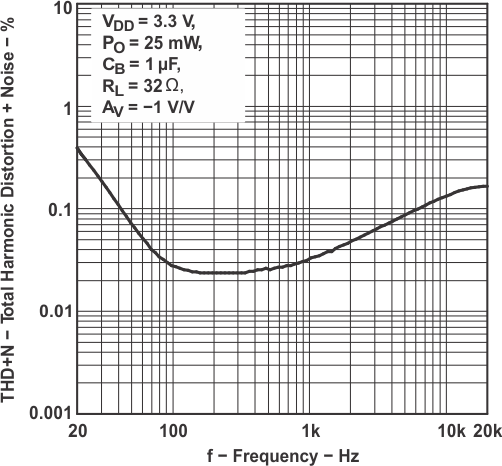Figure 1. Total Harmonic Distortion + Noise vs Frequency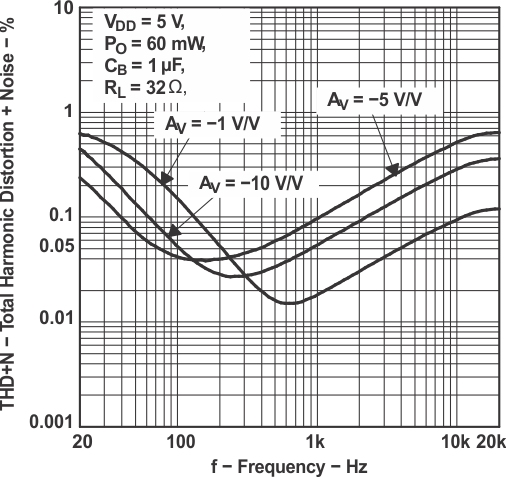Figure 3. Total Harmonic Distortion + Noise vs Frequency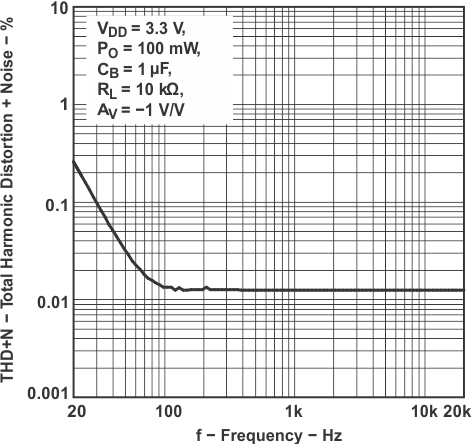Figure 5. Total Harmonic Distortion + Noise vs Frequency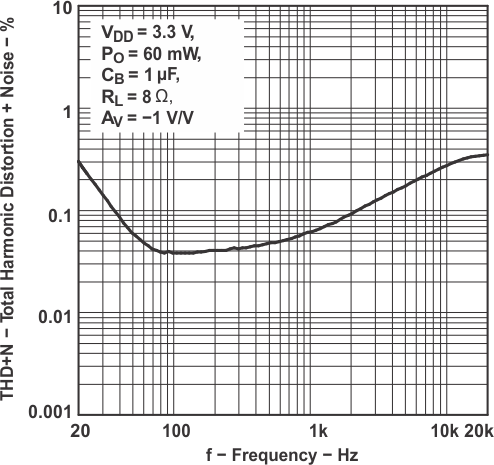Figure 7. Total Harmonic Distortion + Noise vs Frequency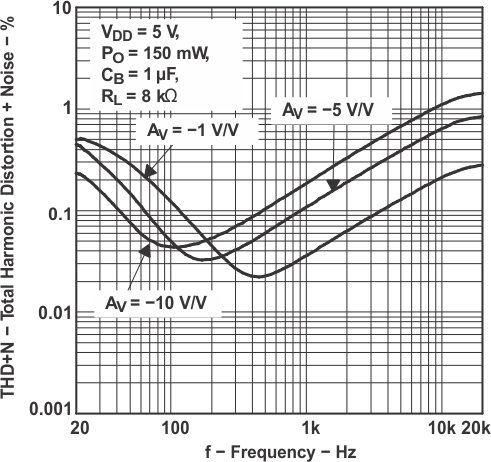Figure 9. Total Harmonic Distortion + Noise vs Frequency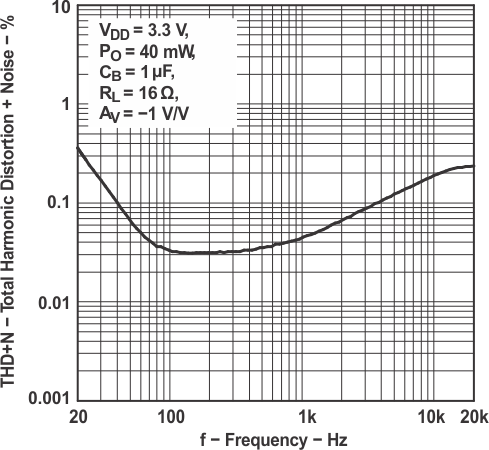Figure 11. Total Harmonic Distortion + Noise vs Frequency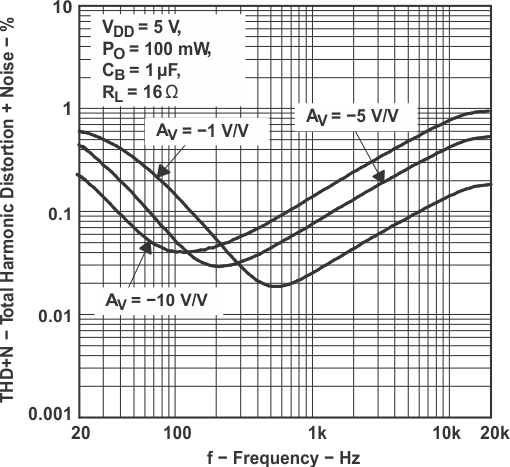Figure 13. Total Harmonic Distortion + Noise vs Frequency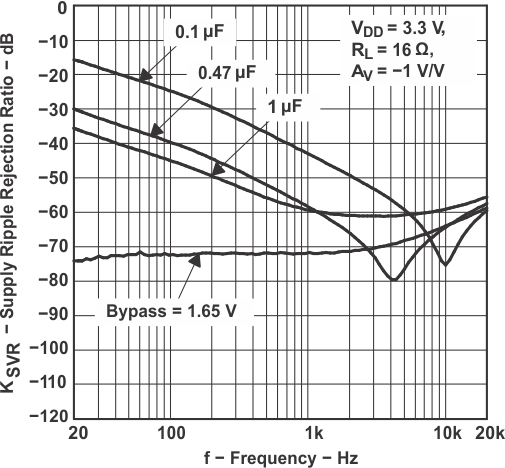Figure 15. Supply Ripple Rejection Ratio vs Frequency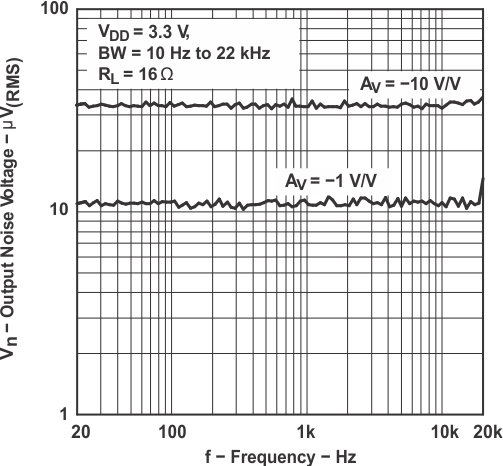Figure 17. Output Noise Voltage vs Frequency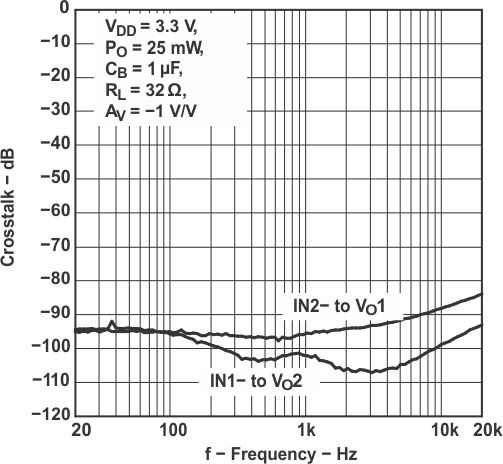Figure 19. Crosstalk vs Frequency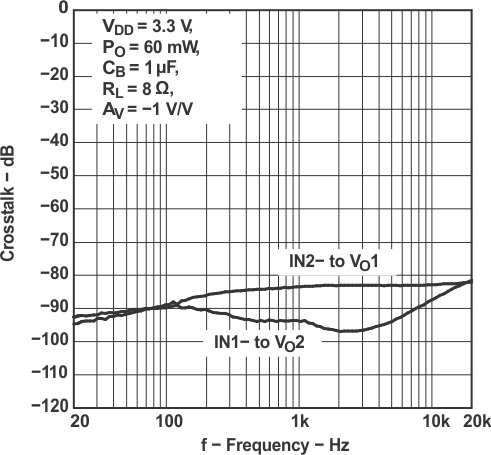Figure 21. Crosstalk vs Frequency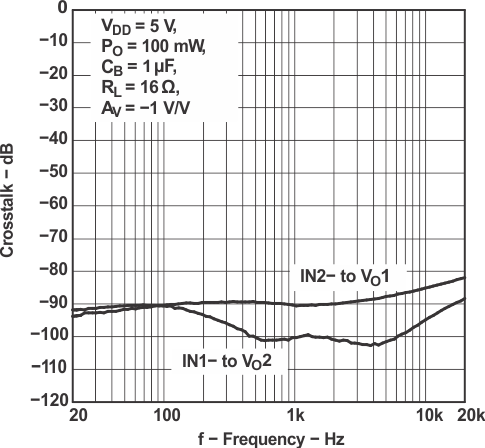Figure 23. Crosstalk vs Frequency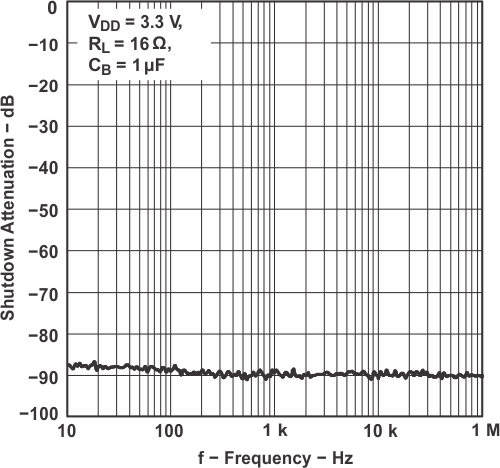Figure 25. Shutdown Attenuation vs Frequency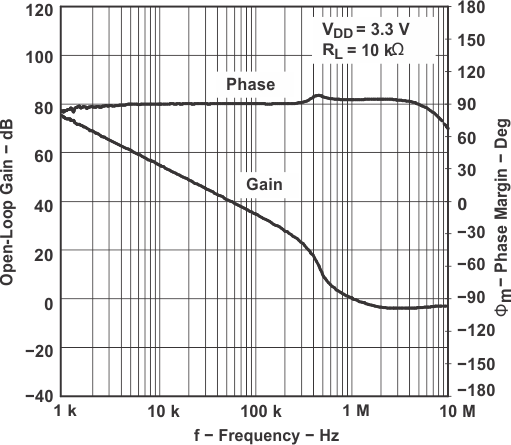Figure 27. Open-Loop Gain and Phase Margin vs Frequency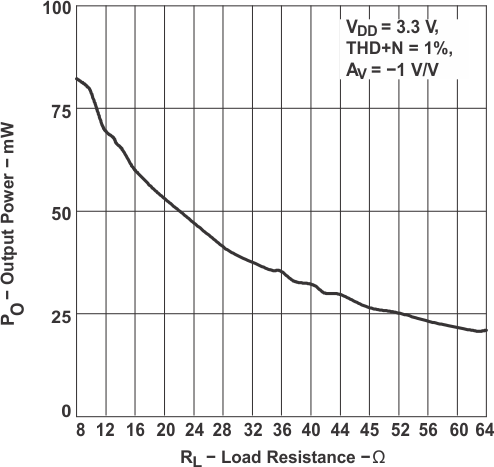Figure 29. Output Power vs Load Resistance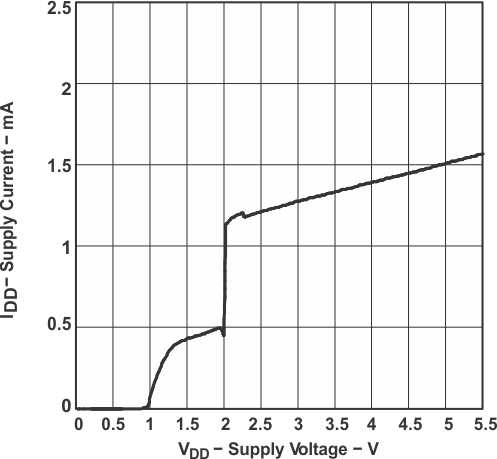Figure 31. Supply Current vs Supply Voltage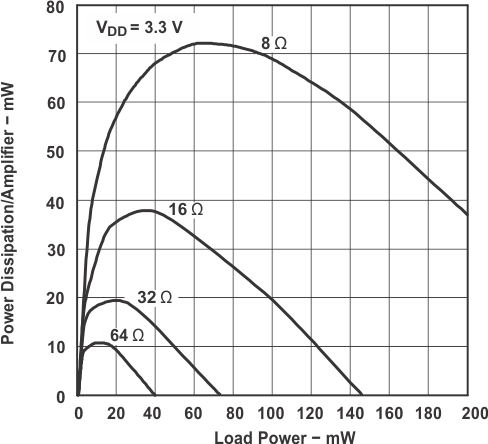Figure 33. Power Dissipation and Amplifier vs Load Power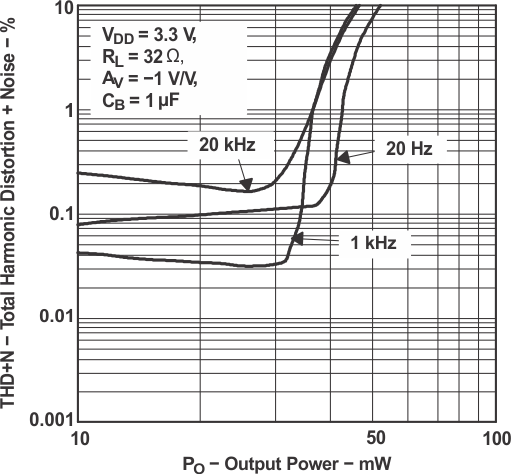Figure 2. Total Harmonic Distortion + Noise vs Output Power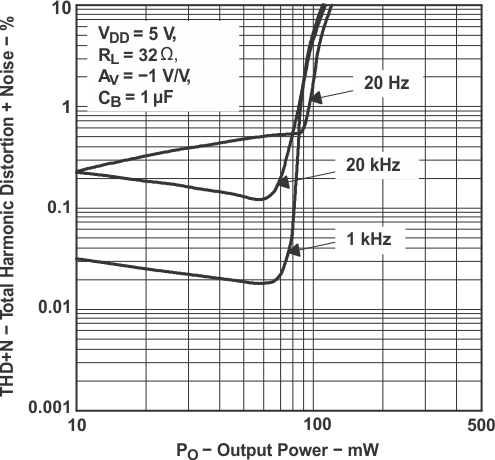Figure 4. Total Harmonic Distortion + Noise vs Output Power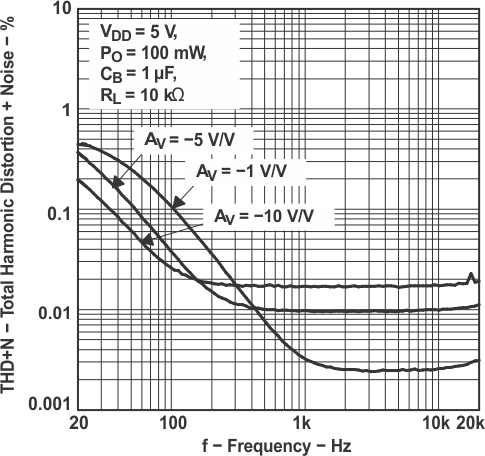Figure 6. Total Harmonic Distortion + Noise vs Frequency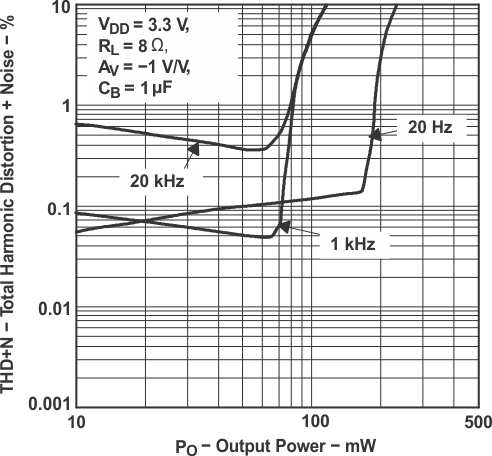Figure 8. Total Harmonic Distortion + Noise vs Output Power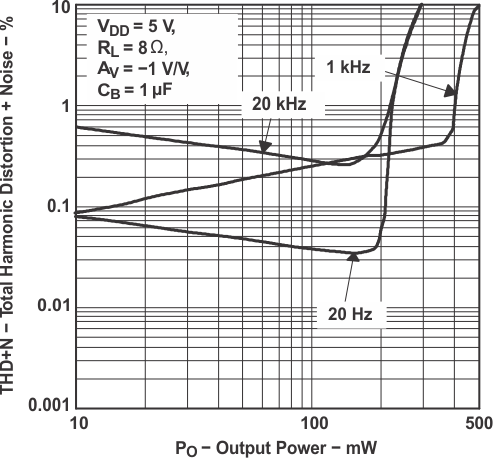Figure 10. Total Harmonic Distortion + Noise vs Output Power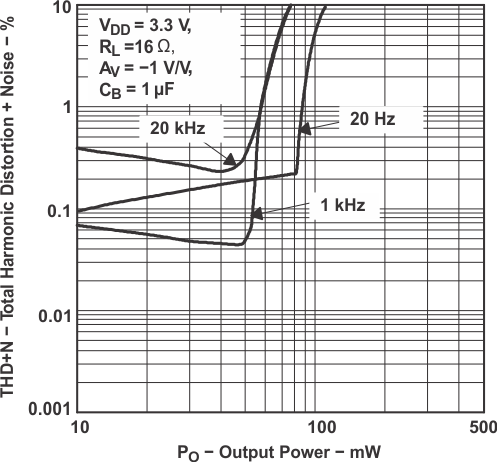Figure 12. Total Harmonic Distortion + Noise vs Output Power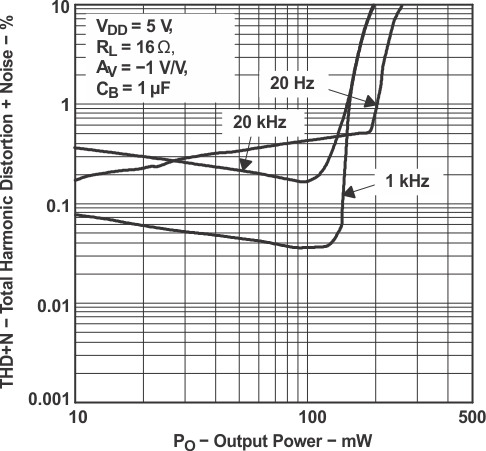Figure 14. Total Harmonic Distortion + Noise vs Output Power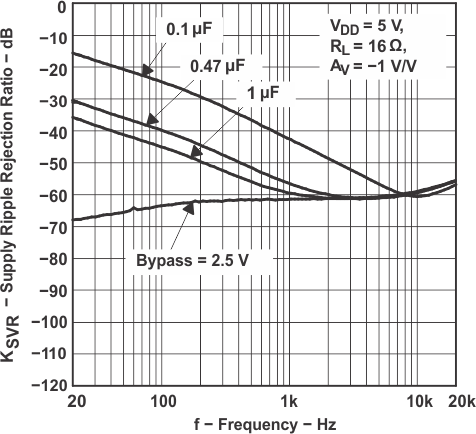Figure 16. Supply Ripple Rejection Ratio vs Frequency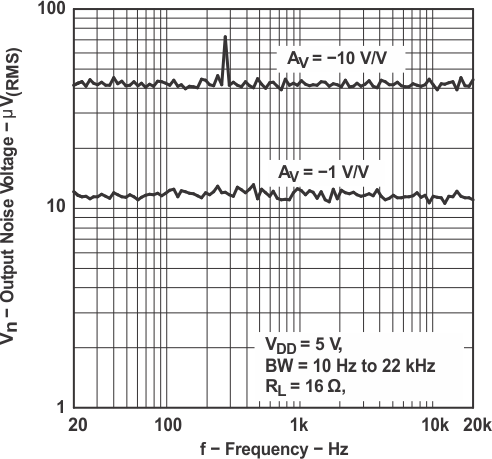Figure 18. Output Noise Voltage vs Frequency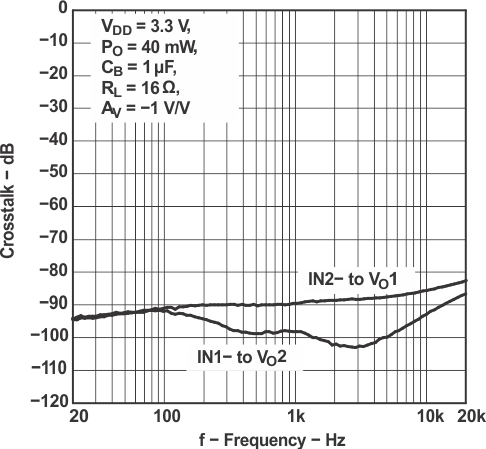Figure 20. Crosstalk vs Frequency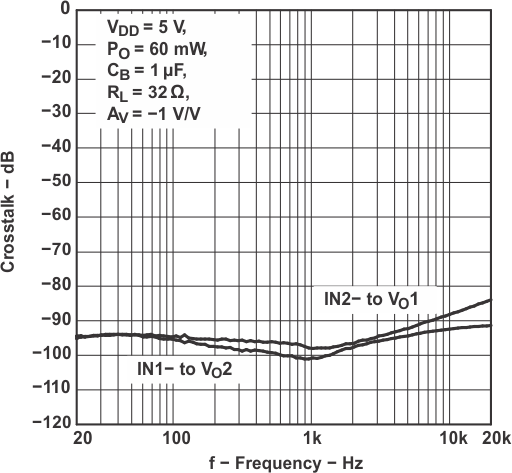Figure 22. Crosstalk vs FrequencyFigure 24. Crosstalk vs Frequency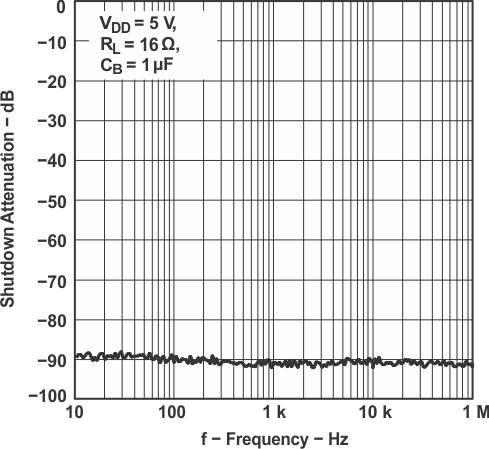Figure 26. Shutdown Attenuation vs Frequency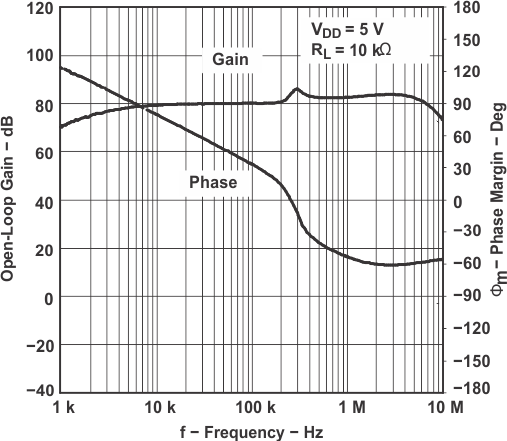Figure 28. Open-Loop Gain and Phase Margin vs Frequency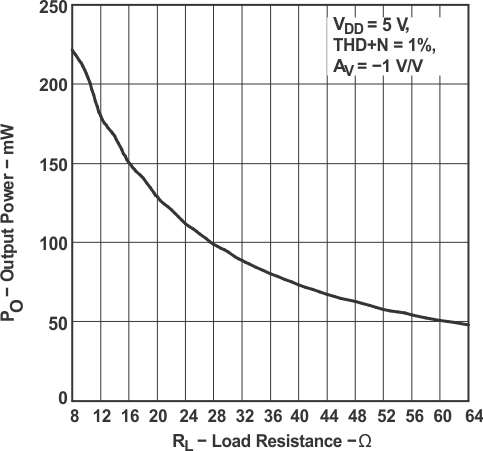Figure 30. Output Power vs Load Resistance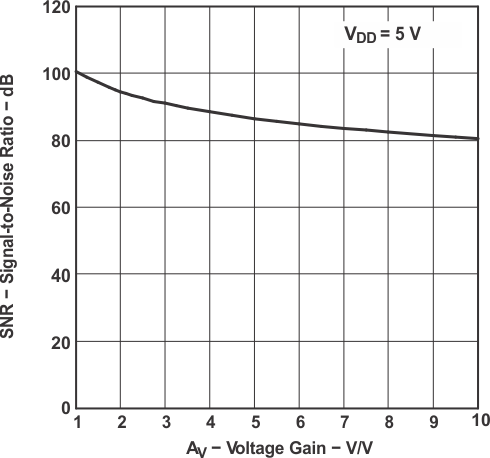Figure 32. Signal-to-Noise Ratio vs Voltage Gain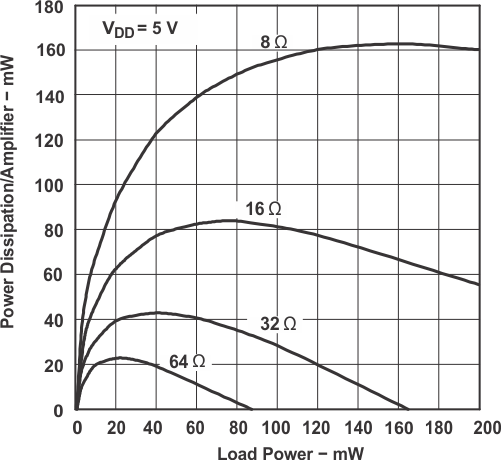Figure 34. Power Dissipation and Amplifier vs Load Power